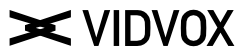#1

Hi,
as an exercise I wish to recreate this pictures.
I have the ring and circles function, and I know that I need to use a
For loop function.

But I’m a bit lost on how to divide and place the circles on the ring !?

Any help would be appreciate.

Best

0 Likes

#2

Or maybe some examples which I can studies ?

0 Likes

#3

Hello!
There are a few ways you can do this! Its a great problem.
You need to read up on polar coordinate system.
something that you can use is this modPolar function from mercury.sexy/hg_sdf

``````// Repeat around the origin by a fixed angle.
// For easier use, num of repetitions is use to specify the angle.
float pModPolar(inout vec2 p, float repetitions) {
float angle = 2*(3.14152)/repetitions;
float a = atan(p.y, p.x) + angle/2.;
float r = length(p);
float c = floor(a/angle);
a = mod(a,angle) - angle/2.;
p = vec2(cos(a), sin(a))*r;
// For an odd number of repetitions, fix cell index of the cell in -x direction
// (cell index would be e.g. -5 and 5 in the two halves of the cell):
if (abs(c) >= (repetitions/2)) c = abs(c);
return c;
}
``````

What this does is it repeats a section of space around a center, creating a kaleidoscope effect. It returns a number unique to the slice, so you can use that to change the color of the circle.
You will have to find where to offset the circle so it can show up in the reflection.
Hopefully you can find this helpful!

2 Likes

#4

I integrated the function in my shaders, but as I get float with this function and need a vec2 for the position of my circle I’m missing an element

And Should I use a loop function somewhere ?

The aim of this shaders is to create an Euclidean Circle like this one : https://www.researchgate.net/figure/The-six-fundamental-African-and-Latin-American-rhythms-which-all-have-equal-sum-of_fig1_237419500

1 Like

#5

So this function uses the `inout` declaration for the position parameter, meaning it changes the vec2 in place. What it returns is a number unique to the slice, so you can use that to change the color of the circle. Do you understand what the modPolar function does? Just checking bc I can help if not :)

This modPolar function method doesnt need a for loop to get the result. Its modding the space i.g. repeating space. But there is a different way to do this that uses a for loop, i personally think its a little harder that way but I could be wrong. You could try it and post your results here & i can look at it.

This problem is not easy- are you working on it to learn GLSL? if you are looking for a way to get that result GLSL isnt the fastest way there. Id recommend a more imperative language like processing, p5js or something like that.

1 Like

#6